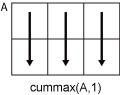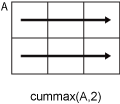# cummax

## 语法

``M = cummax(A)``
``M = cummax(A,dim)``
``M = cummax(___,direction)``
``M = cummax(___,nanflag)``

## 说明

``M = cummax(A)` 返回 `A` 的累积最大元素。默认情况下，`cummax(A)` 沿其大小不为 1 的第一个数组维度运算。如果 `A` 为向量，则 `cummax(A)` 返回一个包含 `A` 的累积最大值的等大小向量。如果 `A` 是矩阵，则 `cummax(A)` 返回一个等大小的矩阵，其中包含 `A` 的各列中的累积最大值。如果 `A` 是多维数组，则 `cummax(A)` 沿 `A` 的大小不为 1 的第一个数组维度返回一个等大小的数组，其中包含累积最大值。`

``M = cummax(A,dim)` 返回沿维度 `dim` 的累积最大值。例如，如果 `A` 是矩阵，则 `cummax(A,2)` 沿 `A` 的各行返回累积最大值。`

``M = cummax(___,direction)` 可选择性地使用上述任何语法指定方向。必须指定 `A`，也可以指定 `dim`。例如，`cummax(A,2,'reverse')` 通过从尾到头计算 `A` 的第二个维度返回 `A` 的累积最大值。`

``M = cummax(___,nanflag)` 指定在上述任意语法的计算中包括还是忽略 `NaN` 值。`cummax(A,'includenan')` 会在计算中包括所有 `NaN` 值，而 `cummax(A,'omitnan')` 则忽略这些值。`

## 示例

`v = randi(10,1,10)`
```v = 1×10 9 10 2 10 7 1 3 6 10 10 ```
`M = cummax(v)`
```M = 1×10 9 10 10 10 10 10 10 10 10 10 ```

`A = [3 5 2; 1 6 3; 7 8 1]`
```A = 3×3 3 5 2 1 6 3 7 8 1 ```
`M = cummax(A)`
```M = 3×3 3 5 2 3 6 3 7 8 3 ```

`A = [3 5 2; 1 6 3; 7 8 1]`
```A = 3×3 3 5 2 1 6 3 7 8 1 ```
`M = cummax(A,2)`
```M = 3×3 3 5 5 1 6 6 7 8 8 ```

`A = cat(3,[1 2; 3 4],[9 10; 11 12],[5 6; 7 8])`
```A = A(:,:,1) = 1 2 3 4 A(:,:,2) = 9 10 11 12 A(:,:,3) = 5 6 7 8 ```
`M = cummax(A,3,'reverse')`
```M = M(:,:,1) = 9 10 11 12 M(:,:,2) = 9 10 11 12 M(:,:,3) = 5 6 7 8 ```

```A = [3 5 NaN 9 0 NaN]; M = cummax(A)```
```M = 1×6 3 5 5 9 9 9 ```

`M = cummax(A,'includenan')`
```M = 1×6 3 5 NaN NaN NaN NaN ```

## 输入参数

• `cummax(A,1)` 处理 `A` 的各列中的连续元素并返回一个与 `A` 等大小的包含各列中的累积最大值的数组。• `cummax(A,2)` 处理 `A` 的各行中的连续元素并返回一个与 `A` 等大小的包含各行中的累积最大值的数组。• `'forward'` 从活动维度的 `1``end` 运算。

• `'reverse'` 从活动维度的 `end``1` 运算。

`NaN` 条件，指定为下列值之一：

• `'omitnan'` - 忽略输入中的所有 `NaN` 值。如果输入有连续前导 `NaN` 值，则 `cummax` 在对应的输出元素中返回 `NaN`。例如，`cummax([NaN 7 13 6],'omitnan')` 返回行向量 `[NaN 7 13 13]`

• `'includenan'` - 计算累积最大值时包括来自输入的 `NaN` 值，在输出中得到 `NaN` 值。

## 提示

• 利用许多累积函数中的 `'reverse'` 选项可以快速执行定向计算，而不需要翻转或反射输入数组。